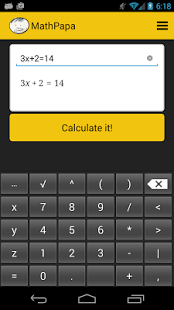MathPapa

Algebra Calculator & Equation Solver App

4.5

• Category Education & Languages
• Program license Free
• Version 1.0.5
• Size 3 MB
• Works under: Android
• Program available in English
• Content rating Everyone
• Package name com.mathpapa.mathpapa
• Program by

There are a ton of learning tools out there that you can download as an app, and MathPapa, for the Android mobile OS, is designed to help people practice and learn algebra.

Overview of MathPapa

As students progress through school and pass geometry and other math courses, they run into algebra eventually, which is far more complex and tougher to master. Luckily there are a lot of tools available today to help people with algebra, like the Android app MathPapa. A cool, lightweight app that deals in math questions, quizzes, games and more, MathPapa is essentially an algebra tutor with which people can practice at their convenience in order to learn the discipline.

This is a free app created by MathPapa for the Android mobile OS, and it's a small package that only weighs in at 3mb. The only available language is English, so it's marketed more toward America and other English speaking nations. It runs smoothly and offers up a ton of different algebra problems and solutions.

How MathPapa Works

Upon initially opening MathPapa, you can choose in which direction you wish to go with the app. You will see an area to calculate equations, an area to take lessons, and an area to practice algebra. It all depends on what you need.

For instance, if you just want to solve an algebra problem, then you simply enter the numbers into the Algebra Calculator section, and you will get an answer. Not only will you get a quick answer, but you will also get the math itself, as in how that answer was reached, so that you can see how it was done. You can also select lessons, which will teach you how to do some algebra problems and reach the proper conclusions. The same holds true for practicing, in that you can work on solving things yourself.

MathPapa Features

The features for MathPapa are basically all in the tabs already explained. You get a calculator that is able to solve most algebra problems you put in. Once you press the “Calculate” button, you'll see a variety of math lines underneath, which explain to you how that conclusion was reached. In the Practice section, what you're getting are a variety of math problems that you will practice solving yourself.

The Lessons feature is basically like a blend of Practice and the calculator, in that you will be shown how to handle the problems and ultimately get a chance to answer them yourself. There are also quizzes in the Lessons section, which present themselves more as math games that could be fun once you get the hang of it all.

Pros and Cons of MathPapa

Pros

• A very accurate calculator
• Fun and easy lessons
• Can teach you algebra quickly

Cons

• Database might not include all algebra answers
• Design is pretty simplistic and dullOther permissions

• ACCESS_NETWORK_STATE: Allows applications to access information about networks.
• INTERNET: Allows applications to open network sockets.
You might also be interested in…

All Math Formula1.6

All Math Formula with Algebra, Geometry, Trigonometry & Calculus

YHomework2.551

The math solver that actually works! No gimmicks-Instant step-by-step solutions!

Symbolab2.11

Math solver with steps: integrals, equations, algebra, trig, calculus and more

Cymath1.31

Enter your math problem, and let Cymath solve it for you step-by-step!

Math Tricks2.4

This program is dedicated to showing you a variety of interesting math tricks

Math Expert3.3

Formula collection of math and physics